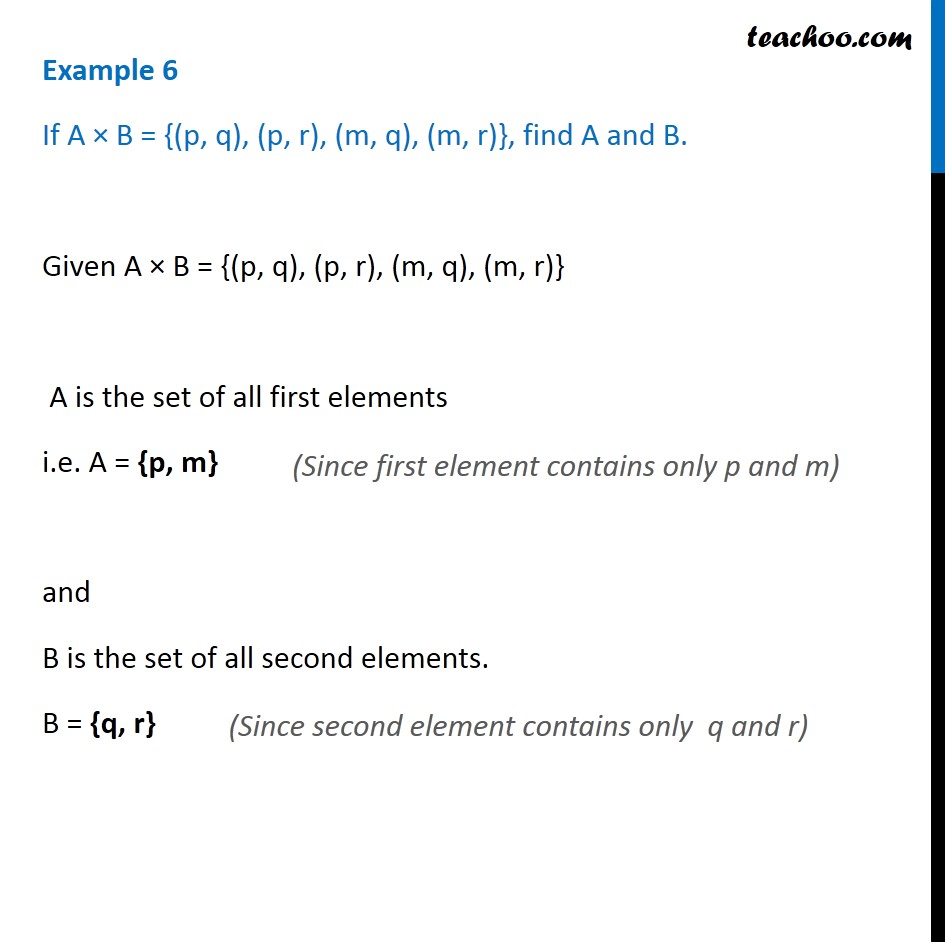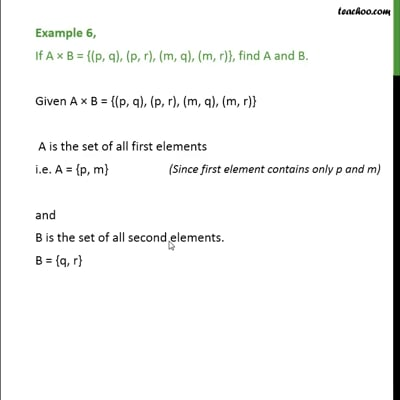Examples

Chapter 2 Class 11 Relations and Functions
Serial order wiseThis video is only available for Teachoo black users

Learn in your speed, with individual attention - Teachoo Maths 1-on-1 Class

### Transcript

Example 6 If A × B = {(p, q), (p, r), (m, q), (m, r)}, find A and B. Given A × B = {(p, q), (p, r), (m, q), (m, r)} A is the set of all first elements i.e. A = {p, m} and B is the set of all second elements. B = {q, r} Example 6 If A × B = {(p, q), (p, r), (m, q), (m, r)}, find A and B. Given A × B = {(p, q), (p, r), (m, q), (m, r)} A is the set of all first elements i.e. A = {p, m} and B is the set of all second elements. B = {q, r} (Since second element contains only q and r)